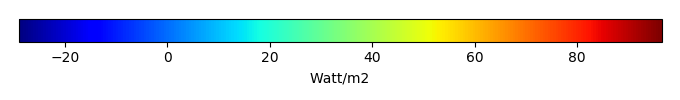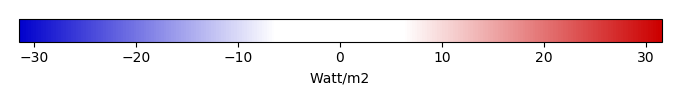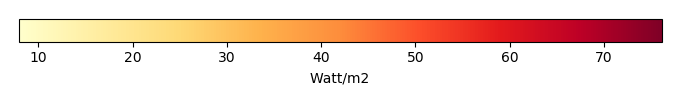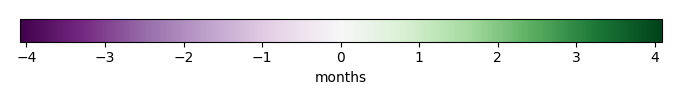# Mean State

Period Mean (original grids) [Watt/m2]
Model Period Mean (intersection) [Watt/m2]
Model Period Mean (complement) [Watt/m2]
Benchmark Period Mean (intersection) [Watt/m2]
Benchmark Period Mean (complement) [Watt/m2]
Bias [Watt/m2]
RMSE [Watt/m2]
Phase Shift [months]
Bias Score 
RMSE Score 
Seasonal Cycle Score 
Spatial Distribution Score 
Overall Score 
Benchmark [-] 44.1
CLM4 [-] 44.9 44.2 57.8 44.2 12.4 0.430 24.4 1.42 0.33 0.15 0.79 0.69 0.42
CLM4.5 [-] 52.4 51.5 63.6 44.2 12.4 7.70 18.2 1.48 0.42 0.22 0.78 0.83 0.49
CLM5 [-] 51.8 51.1 64.4 44.2 12.4 7.31 18.6 1.46 0.41 0.24 0.79 0.76 0.49
Period Mean (original grids) [Watt/m2]
Model Period Mean (intersection) [Watt/m2]
Model Period Mean (complement) [Watt/m2]
Benchmark Period Mean (intersection) [Watt/m2]
Benchmark Period Mean (complement) [Watt/m2]
Bias [Watt/m2]
RMSE [Watt/m2]
Phase Shift [months]
Bias Score 
RMSE Score 
Seasonal Cycle Score 
Spatial Distribution Score 
Overall Score 
Benchmark [-] 57.5
CLM4 [-] 55.9 56.0 84.3 57.5 20.8 -1.35 20.8 0.916 0.53 0.38 0.90 0.96 0.63
CLM4.5 [-] 56.8 57.0 89.4 57.5 20.8 -0.402 20.4 1.05 0.55 0.38 0.88 0.97 0.63
CLM5 [-] 59.0 59.1 89.7 57.5 20.8 1.68 21.6 1.33 0.53 0.36 0.85 0.97 0.61
Period Mean (original grids) [Watt/m2]
Model Period Mean (intersection) [Watt/m2]
Model Period Mean (complement) [Watt/m2]
Benchmark Period Mean (intersection) [Watt/m2]
Benchmark Period Mean (complement) [Watt/m2]
Bias [Watt/m2]
RMSE [Watt/m2]
Phase Shift [months]
Bias Score 
RMSE Score 
Seasonal Cycle Score 
Spatial Distribution Score 
Overall Score 
Benchmark [-] 52.8
CLM4 [-] 56.0 55.8 62.2 53.0 19.6 3.12 19.8 0.619 0.58 0.41 0.94 0.95 0.66
CLM4.5 [-] 56.7 56.5 64.3 53.0 19.6 3.86 18.4 0.632 0.61 0.44 0.94 0.97 0.68
CLM5 [-] 58.2 57.9 66.6 53.0 19.6 5.27 19.2 0.627 0.56 0.46 0.94 0.93 0.67
Period Mean (original grids) [Watt/m2]
Model Period Mean (intersection) [Watt/m2]
Model Period Mean (complement) [Watt/m2]
Benchmark Period Mean (intersection) [Watt/m2]
Benchmark Period Mean (complement) [Watt/m2]
Bias [Watt/m2]
RMSE [Watt/m2]
Phase Shift [months]
Bias Score 
RMSE Score 
Seasonal Cycle Score 
Spatial Distribution Score 
Overall Score 
Benchmark [-] 18.0
CLM4 [-] 3.35 3.62 -0.834 18.1 5.64 -14.7 25.0 0.781 0.55 0.46 0.94 0.80 0.64
CLM4.5 [-] 3.40 3.67 -1.25 18.1 5.64 -14.8 23.0 0.691 0.55 0.51 0.95 0.80 0.66
CLM5 [-] -0.719 -0.431 -5.58 18.1 5.64 -18.8 25.0 0.610 0.46 0.55 0.95 0.52 0.61
Period Mean (original grids) [Watt/m2]
Model Period Mean (intersection) [Watt/m2]
Model Period Mean (complement) [Watt/m2]
Benchmark Period Mean (intersection) [Watt/m2]
Benchmark Period Mean (complement) [Watt/m2]
Bias [Watt/m2]
RMSE [Watt/m2]
Phase Shift [months]
Bias Score 
RMSE Score 
Seasonal Cycle Score 
Spatial Distribution Score 
Overall Score 
Benchmark [-] 22.1
CLM4 [-] 20.7 19.1 28.6 22.8 10.8 -0.765 19.8 1.56 0.24 0.13 0.78 0.46 0.35
CLM4.5 [-] 40.4 39.5 44.6 22.8 10.8 18.5 25.5 1.41 0.13 0.12 0.80 0.66 0.37
CLM5 [-] 29.9 28.6 36.4 22.8 10.8 8.44 22.3 1.78 0.13 0.14 0.73 0.42 0.31
Period Mean (original grids) [Watt/m2]
Model Period Mean (intersection) [Watt/m2]
Model Period Mean (complement) [Watt/m2]
Benchmark Period Mean (intersection) [Watt/m2]
Benchmark Period Mean (complement) [Watt/m2]
Bias [Watt/m2]
RMSE [Watt/m2]
Phase Shift [months]
Bias Score 
RMSE Score 
Seasonal Cycle Score 
Spatial Distribution Score 
Overall Score 
Benchmark [-] 27.0
CLM4 [-] 21.8 21.9 17.1 27.1 8.81 -5.09 16.9 0.497 0.76 0.57 0.96 0.97 0.77
CLM4.5 [-] 17.0 17.1 18.0 27.1 8.81 -9.91 19.0 0.982 0.65 0.57 0.90 0.95 0.73
CLM5 [-] 10.9 10.9 15.3 27.1 8.81 -16.1 22.4 0.994 0.54 0.58 0.90 0.88 0.69
Period Mean (original grids) [Watt/m2]
Model Period Mean (intersection) [Watt/m2]
Model Period Mean (complement) [Watt/m2]
Benchmark Period Mean (intersection) [Watt/m2]
Benchmark Period Mean (complement) [Watt/m2]
Bias [Watt/m2]
RMSE [Watt/m2]
Phase Shift [months]
Bias Score 
RMSE Score 
Seasonal Cycle Score 
Spatial Distribution Score 
Overall Score 
Benchmark [-] 28.4
CLM4 [-] 36.3 36.0 39.4 28.4 24.4 7.48 20.3 0.981 0.67 0.47 0.88 0.91 0.68
CLM4.5 [-] 35.0 34.5 40.5 28.4 24.4 5.98 21.0 1.01 0.66 0.45 0.88 0.90 0.67
CLM5 [-] 35.2 34.7 40.6 28.4 24.4 6.23 20.7 1.05 0.67 0.47 0.87 0.92 0.68
Period Mean (original grids) [Watt/m2]
Model Period Mean (intersection) [Watt/m2]
Model Period Mean (complement) [Watt/m2]
Benchmark Period Mean (intersection) [Watt/m2]
Benchmark Period Mean (complement) [Watt/m2]
Bias [Watt/m2]
RMSE [Watt/m2]
Phase Shift [months]
Bias Score 
RMSE Score 
Seasonal Cycle Score 
Spatial Distribution Score 
Overall Score 
Benchmark [-] 40.3
CLM4 [-] 48.8 48.8 51.1 40.6 11.0 7.34 23.6 0.524 0.55 0.38 0.96 0.90 0.64
CLM4.5 [-] 53.3 53.3 56.9 40.6 11.0 12.1 24.2 0.528 0.52 0.40 0.96 0.95 0.65
CLM5 [-] 52.0 52.0 56.2 40.6 11.0 10.9 23.0 0.686 0.54 0.43 0.94 0.94 0.65
Period Mean (original grids) [Watt/m2]
Model Period Mean (intersection) [Watt/m2]
Model Period Mean (complement) [Watt/m2]
Benchmark Period Mean (intersection) [Watt/m2]
Benchmark Period Mean (complement) [Watt/m2]
Bias [Watt/m2]
RMSE [Watt/m2]
Phase Shift [months]
Bias Score 
RMSE Score 
Seasonal Cycle Score 
Spatial Distribution Score 
Overall Score 
Benchmark [-] 15.2
CLM4 [-] 0.805 1.30 -7.30 15.2 3.78 -14.2 24.5 0.934 0.57 0.46 0.93 0.67 0.62
CLM4.5 [-] -2.17 -1.72 -9.51 15.2 3.78 -17.2 24.2 1.01 0.50 0.52 0.91 0.46 0.58
CLM5 [-] -9.16 -8.77 -15.8 15.2 3.78 -24.2 28.4 0.787 0.38 0.57 0.94 0.20 0.53
Period Mean (original grids) [Watt/m2]
Model Period Mean (intersection) [Watt/m2]
Model Period Mean (complement) [Watt/m2]
Benchmark Period Mean (intersection) [Watt/m2]
Benchmark Period Mean (complement) [Watt/m2]
Bias [Watt/m2]
RMSE [Watt/m2]
Phase Shift [months]
Bias Score 
RMSE Score 
Seasonal Cycle Score 
Spatial Distribution Score 
Overall Score 
Benchmark [-] 56.5
CLM4 [-] 63.6 64.2 51.3 56.9 15.0 6.74 19.7 0.712 0.71 0.50 0.94 0.97 0.72
CLM4.5 [-] 63.9 64.3 54.1 56.9 15.0 7.00 20.4 0.717 0.71 0.49 0.94 0.94 0.71
CLM5 [-] 64.1 64.8 51.4 56.9 15.0 7.25 18.2 0.602 0.70 0.56 0.95 0.98 0.75
Period Mean (original grids) [Watt/m2]
Model Period Mean (intersection) [Watt/m2]
Model Period Mean (complement) [Watt/m2]
Benchmark Period Mean (intersection) [Watt/m2]
Benchmark Period Mean (complement) [Watt/m2]
Bias [Watt/m2]
RMSE [Watt/m2]
Phase Shift [months]
Bias Score 
RMSE Score 
Seasonal Cycle Score 
Spatial Distribution Score 
Overall Score 
Benchmark [-] 20.2
CLM4 [-] 27.3 27.2 29.5 20.4 7.08 7.03 21.4 0.578 0.67 0.48 0.94 0.74 0.66
CLM4.5 [-] 26.5 26.4 29.4 20.4 7.08 6.26 21.8 0.506 0.67 0.47 0.94 0.70 0.65
CLM5 [-] 21.2 21.0 25.6 20.4 7.08 1.11 22.2 0.696 0.68 0.47 0.92 0.58 0.62
Period Mean (original grids) [Watt/m2]
Model Period Mean (intersection) [Watt/m2]
Model Period Mean (complement) [Watt/m2]
Benchmark Period Mean (intersection) [Watt/m2]
Benchmark Period Mean (complement) [Watt/m2]
Bias [Watt/m2]
RMSE [Watt/m2]
Phase Shift [months]
Bias Score 
RMSE Score 
Seasonal Cycle Score 
Spatial Distribution Score 
Overall Score 
Benchmark [-] 34.3
CLM4 [-] 33.0 38.0 12.6 34.6 11.5 3.29 21.9 0.886 0.59 0.43 0.91 0.87 0.65
CLM4.5 [-] 34.3 39.3 13.9 34.6 11.5 4.63 22.3 0.954 0.57 0.43 0.90 0.86 0.64
CLM5 [-] 32.2 37.2 11.2 34.6 11.5 2.60 22.5 1.02 0.56 0.46 0.89 0.79 0.63
Period Mean (original grids) [Watt/m2]
Model Period Mean (intersection) [Watt/m2]
Model Period Mean (complement) [Watt/m2]
Benchmark Period Mean (intersection) [Watt/m2]
Benchmark Period Mean (complement) [Watt/m2]
Bias [Watt/m2]
RMSE [Watt/m2]
Phase Shift [months]
Bias Score 
RMSE Score 
Seasonal Cycle Score 
Spatial Distribution Score 
Overall Score 
Benchmark [-] 22.7
CLM4 [-] 55.9 56.9 55.0 22.8 18.3 34.8 43.4 1.16 0.50 0.46 0.95 0.79 0.63
CLM4.5 [-] 56.4 57.5 55.3 22.8 18.3 35.5 44.2 1.12 0.50 0.44 0.95 0.79 0.63
CLM5 [-] 56.4 58.3 54.6 22.8 18.3 36.2 44.1 1.34 0.51 0.45 0.93 0.80 0.63
Period Mean (original grids) [Watt/m2]
Model Period Mean (intersection) [Watt/m2]
Model Period Mean (complement) [Watt/m2]
Benchmark Period Mean (intersection) [Watt/m2]
Benchmark Period Mean (complement) [Watt/m2]
Bias [Watt/m2]
RMSE [Watt/m2]
Phase Shift [months]
Bias Score 
RMSE Score 
Seasonal Cycle Score 
Spatial Distribution Score 
Overall Score 
Benchmark [-] 17.0
CLM4 [-] 6.67 7.42 -5.67 17.0 4.51 -10.3 21.2 0.638 0.68 0.53 0.96 0.75 0.69
CLM4.5 [-] 4.51 5.11 -5.87 17.0 4.51 -12.4 21.4 0.814 0.62 0.55 0.93 0.71 0.67
CLM5 [-] -1.36 -0.796 -10.9 17.0 4.51 -18.3 25.6 1.08 0.50 0.55 0.89 0.49 0.59
Period Mean (original grids) [Watt/m2]
Model Period Mean (intersection) [Watt/m2]
Model Period Mean (complement) [Watt/m2]
Benchmark Period Mean (intersection) [Watt/m2]
Benchmark Period Mean (complement) [Watt/m2]
Bias [Watt/m2]
RMSE [Watt/m2]
Phase Shift [months]
Bias Score 
RMSE Score 
Seasonal Cycle Score 
Spatial Distribution Score 
Overall Score 
Benchmark [-] 47.7
CLM4 [-] 53.2 52.6 59.9 48.2 15.9 4.69 25.1 0.534 0.46 0.50 0.96 0.69 0.62
CLM4.5 [-] 54.0 53.2 62.3 48.2 15.9 5.51 26.8 0.493 0.45 0.48 0.96 0.67 0.61
CLM5 [-] 54.1 53.3 62.2 48.2 15.9 5.59 24.9 0.650 0.46 0.52 0.95 0.68 0.63
Period Mean (original grids) [Watt/m2]
Model Period Mean (intersection) [Watt/m2]
Model Period Mean (complement) [Watt/m2]
Benchmark Period Mean (intersection) [Watt/m2]
Benchmark Period Mean (complement) [Watt/m2]
Bias [Watt/m2]
RMSE [Watt/m2]
Phase Shift [months]
Bias Score 
RMSE Score 
Seasonal Cycle Score 
Spatial Distribution Score 
Overall Score 
Benchmark [-] 28.6
CLM4 [-] 32.1 32.3 28.0 28.7 12.1 3.73 15.8 1.42 0.69 0.43 0.79 0.92 0.65
CLM4.5 [-] 31.3 31.4 31.4 28.7 12.1 2.84 17.6 2.19 0.68 0.38 0.67 0.93 0.60
CLM5 [-] 31.5 31.5 33.6 28.7 12.1 3.05 17.9 1.89 0.63 0.40 0.70 0.83 0.59
Period Mean (original grids) [Watt/m2]
Model Period Mean (intersection) [Watt/m2]
Model Period Mean (complement) [Watt/m2]
Benchmark Period Mean (intersection) [Watt/m2]
Benchmark Period Mean (complement) [Watt/m2]
Bias [Watt/m2]
RMSE [Watt/m2]
Phase Shift [months]
Bias Score 
RMSE Score 
Seasonal Cycle Score 
Spatial Distribution Score 
Overall Score 
Benchmark [-] 26.7
CLM4 [-] 29.9 30.2 17.3 26.9 8.98 2.89 17.1 0.511 0.77 0.58 0.96 0.93 0.76
CLM4.5 [-] 25.3 25.4 20.2 26.9 8.98 -1.72 15.4 0.686 0.78 0.63 0.94 0.97 0.79
CLM5 [-] 17.4 17.4 17.9 26.9 8.98 -9.57 19.3 1.01 0.66 0.60 0.89 0.95 0.74
Period Mean (original grids) [Watt/m2]
Model Period Mean (intersection) [Watt/m2]
Model Period Mean (complement) [Watt/m2]
Benchmark Period Mean (intersection) [Watt/m2]
Benchmark Period Mean (complement) [Watt/m2]
Bias [Watt/m2]
RMSE [Watt/m2]
Phase Shift [months]
Bias Score 
RMSE Score 
Seasonal Cycle Score 
Spatial Distribution Score 
Overall Score 
Benchmark [-] 49.7
CLM4 [-] 38.9 38.9 60.0 49.8 11.0 -10.8 19.0 0.577 0.64 0.66 0.95 0.89 0.76
CLM4.5 [-] 37.0 37.0 64.1 49.8 11.0 -12.7 21.3 0.587 0.60 0.64 0.95 0.93 0.75
CLM5 [-] 37.7 37.8 60.2 49.8 11.0 -11.9 19.2 0.577 0.64 0.66 0.95 0.92 0.76
Period Mean (original grids) [Watt/m2]
Model Period Mean (intersection) [Watt/m2]
Model Period Mean (complement) [Watt/m2]
Benchmark Period Mean (intersection) [Watt/m2]
Benchmark Period Mean (complement) [Watt/m2]
Bias [Watt/m2]
RMSE [Watt/m2]
Phase Shift [months]
Bias Score 
RMSE Score 
Seasonal Cycle Score 
Spatial Distribution Score 
Overall Score 
Benchmark [-] 30.2
CLM4 [-] 26.9 26.7 48.0 30.3 13.0 -2.93 17.7 1.52 0.38 0.17 0.80 0.48 0.40
CLM4.5 [-] 44.3 44.3 53.8 30.3 13.0 14.3 20.5 1.33 0.24 0.18 0.83 0.83 0.45
CLM5 [-] 36.5 36.4 50.4 30.3 13.0 6.57 16.1 1.45 0.33 0.19 0.81 0.70 0.45
Period Mean (original grids) [Watt/m2]
Model Period Mean (intersection) [Watt/m2]
Model Period Mean (complement) [Watt/m2]
Benchmark Period Mean (intersection) [Watt/m2]
Benchmark Period Mean (complement) [Watt/m2]
Bias [Watt/m2]
RMSE [Watt/m2]
Phase Shift [months]
Bias Score 
RMSE Score 
Seasonal Cycle Score 
Spatial Distribution Score 
Overall Score 
Benchmark [-] 40.3
CLM4 [-] 43.7 43.8 44.0 40.4 9.36 3.57 17.7 1.12 0.55 0.36 0.88 0.80 0.59
CLM4.5 [-] 46.4 46.5 45.9 40.4 9.36 6.26 16.7 1.15 0.58 0.37 0.88 0.89 0.62
CLM5 [-] 46.8 47.0 43.6 40.4 9.36 6.69 16.7 0.967 0.51 0.42 0.90 0.78 0.61
Period Mean (original grids) [Watt/m2]
Model Period Mean (intersection) [Watt/m2]
Model Period Mean (complement) [Watt/m2]
Benchmark Period Mean (intersection) [Watt/m2]
Benchmark Period Mean (complement) [Watt/m2]
Bias [Watt/m2]
RMSE [Watt/m2]
Phase Shift [months]
Bias Score 
RMSE Score 
Seasonal Cycle Score 
Spatial Distribution Score 
Overall Score 
Benchmark [-] 41.5
CLM4 [-] 57.4 56.9 61.6 42.0 6.56 13.7 28.1 0.839 0.61 0.50 0.91 0.94 0.69
CLM4.5 [-] 56.1 55.7 61.5 42.0 6.56 12.9 27.8 0.823 0.61 0.51 0.91 0.93 0.69
CLM5 [-] 56.9 56.4 61.4 42.0 6.56 13.2 26.8 0.781 0.61 0.55 0.93 0.94 0.72

# Temporally integrated period mean

BENCHMARK MEANMODEL MEANBIASBIAS SCORERMSERMSE SCOREBENCHMARK MAX MONTHMODEL MAX MONTHDIFFERENCE IN MAX MONTHSEASONAL CYCLE SCORESPATIAL TAYLOR DIAGRAMMODEL COLORS# Spatially integrated regional mean

MODEL COLORSREGIONAL MEANANNUAL CYCLEMONTHLY ANOMALYANNUAL CYCLE# All Models

BenchmarkCLM4CLM4.5CLM5# Data Information

creation_date: Tue Jul 28 15:54:11 PDT 2015

source_file: This product is generated from monthly Global Biosphere Amtospher Flux (GBAF) observations

title: derived GBAF in 0.5x0.5

Approach: I read this variable from data file, and then save the data in NetCDF format by each month and each year. Meanwhile, I also converted the unit from MJoule*m-2*d-1 to Watt/m2.

Temporal resolution: monthly

General information: This product was derived from Monthly estimates of global biosphere-atmosphere fluxes from Biogeochemistry group at Max Planck Institute in Jena, Germany.

Spatial resolution: 0.5x0.5 degree